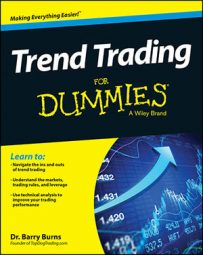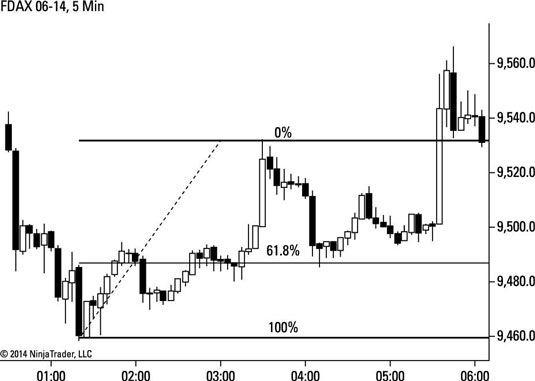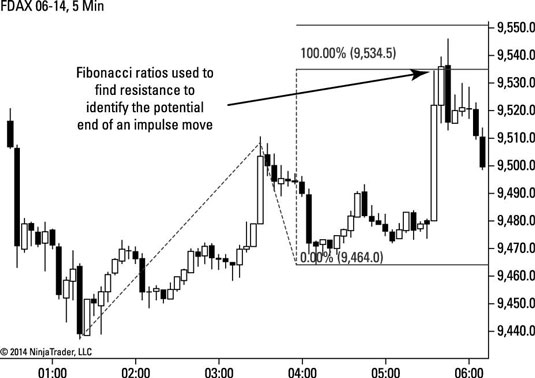##### Trend Trading For DummiesAfter developing his Elliott wave theory, Ralph Nelson Elliott observed that the wave patterns relate to the Fibonacci sequence. The Fibonacci sequence is a series of numbers created by adding the sum of the previous two numbers to create the next number in the sequence:

1 + 2 = 3
2 + 3 = 5
3 + 5 = 8
5 + 8 = 13
8 + 13 = 21
13 + 21 = 34

Thus, the beginning of the sequence is 1, 2, 3, 5, 8, 13, 21, 34. . . .

In trading, Fibonacci ratios are more commonly used than the Fibonacci numbers themselves. You create these ratios by dividing one number in the sequence by another. The most significant of those ratios is the golden ratio, which you get (or get very close to) by dividing any number in the sequence by the number immediately preceding it. For example, 34/21 = 1.619. The actual golden ratio is 1.618.

You use the Fibonacci ratios in conjunction with Elliott waves as potential price levels for impulse and correction moves to begin and end.

The most common Fibonacci ratios used in trading are

0.236
0.352
0.500
0.618
0.786

You use these ratios to measure corrective movements. The figure demonstrates how these ratios help find a support level for a corrective move before the next impulse move begins.Credit: Figure by Barry Burns

For impulse moves, you use those same ratios, but you add a 1, 2, or 3 in front of them:

1.236
1.352
1.500
1.618
1.786

These ratios help measure impulse waves. The following figure shows how these ratios find potential resistance for the end of an impulse wave.Credit: Figure by Barry Burns